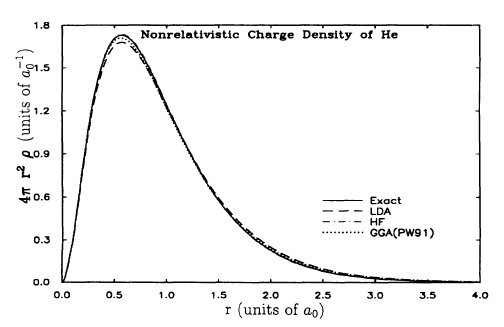# Atomic Radius of H & He: Why is H Larger?

http://www.wacklepedia.com/h/hy/hydrogen.html [Broken]

Question is, why is calculated atomic radius of hydrogen (53 pm) LARGER than calculated radius of helium (31 pm) ? How can a single proton have larger radius than helium which has two proton ?

Also, why nobody yet measure the atomic radius of helium, why it still only 'calculated'--seems like a Ph.D. dissertation project that should have been done years ago ?

Last edited by a moderator:
Well, first, the size of the nucleus has almost zero impact on the "size" of the atom, which is due to the number of electrons.

Second, as I explained recently in this post in a thread farther down, the exact 'radius' of an atom is a notoriously difficult to define concept. They're not tiny hard spheres!

The answer to why helium is smaller is that both of helium's electrons are in the same orbital as the single electron of hydrogen (the 1s orbital), so it's radius does not expand much from having the second electron. However, the doubled nuclear charge causes both electrons to move closer to the nucleus.

Here's what the radial charge distribution looks like for hydrogen: (in units of Bohr radiuses)

And for helium (same scale):As you can see, it's very nearly the same curve, only the helium one has been 'compressed', radially.

Both of these curves are calculated (the helium compares some approximate methods to the exact value). The reason why the values are calculated is simple: Atomic radii can be calculated much more exactly than they can be measured.

The radius of atoms only really increases substantially when you start a new shell, e.g. the next step, lithium, is quite dramatic. Which is why most metal atoms (d-orbital elements) have about the same radius. And can therefore mix with each other in crystals and form alloys.

Last edited by a moderator:
Note in the first plot above that the radial wave function for a hydrogen-like atom is of the form
e-2Zr/a0
where a0 is a fundamental constant = Bohr radius = 529 pm. So the "Bohr radius" of an atom of charge Z is 539/Z p m.